# Working with sensor locations#

This tutorial describes how to read and plot sensor locations, and how MNE-Python handles physical locations of sensors.

As usual we’ll start by importing the modules we need and loading some example data:

```import os
import numpy as np
import matplotlib.pyplot as plt

# the following import is required for matplotlib < 3.2:
from mpl_toolkits.mplot3d import Axes3D  # noqa
import mne

sample_data_folder = mne.datasets.sample.data_path()
sample_data_raw_file = os.path.join(sample_data_folder, 'MEG', 'sample',
'sample_audvis_raw.fif')
```

`Montages` contain sensor positions in 3D (x, y, z in meters), which can be assigned to existing EEG/MEG data. By specifying the locations of sensors relative to the brain, `Montages` play an important role in computing the forward solution and inverse estimates.

In contrast, `Layouts` are idealized 2D representations of sensor positions. They are primarily used for arranging individual sensor subplots in a topoplot or for showing the approximate relative arrangement of sensors as seen from above.

## Working with built-in montages#

The 3D coordinates of MEG sensors are included in the raw recordings from MEG systems. They are automatically stored in the `info` attribute of the `Raw` object upon loading. EEG electrode locations are much more variable because of differences in head shape. Idealized montages for many EEG systems are included in MNE-Python; these files are stored in your `mne-python` directory in the `mne/channels/data/montages` folder:

```montage_dir = os.path.join(os.path.dirname(mne.__file__),
'channels', 'data', 'montages')
print('\nBUILT-IN MONTAGE FILES')
print('======================')
print(sorted(os.listdir(montage_dir)))
```
```BUILT-IN MONTAGE FILES
======================
['EGI_256.csd', 'GSN-HydroCel-128.sfp', 'GSN-HydroCel-129.sfp', 'GSN-HydroCel-256.sfp', 'GSN-HydroCel-257.sfp', 'GSN-HydroCel-32.sfp', 'GSN-HydroCel-64_1.0.sfp', 'GSN-HydroCel-65_1.0.sfp', 'artinis-brite23.elc', 'artinis-octamon.elc', 'biosemi128.txt', 'biosemi16.txt', 'biosemi160.txt', 'biosemi256.txt', 'biosemi32.txt', 'biosemi64.txt', 'easycap-M1.txt', 'easycap-M10.txt', 'mgh60.elc', 'mgh70.elc', 'standard_1005.elc', 'standard_1020.elc', 'standard_alphabetic.elc', 'standard_postfixed.elc', 'standard_prefixed.elc', 'standard_primed.elc']
```

These built-in EEG montages can be loaded with `mne.channels.make_standard_montage` (note that you need to provide the filename without its extension):

```ten_twenty_montage = mne.channels.make_standard_montage('standard_1020')
print(ten_twenty_montage)
```
```<DigMontage | 0 extras (headshape), 0 HPIs, 3 fiducials, 94 channels>
```

Once loaded, a montage can be applied to data with the `set_montage` method, for example `raw.set_montage`. It is also possible to skip the loading step by passing the filename string directly to the `set_montage` method. This will not work with our sample data, because its channel names do not match the channel names in the standard 10–20 montage. Therefore, we do not run the following commands here:

```# these will be equivalent:
# raw_1020 = raw.copy().set_montage(ten_twenty_montage)
# raw_1020 = raw.copy().set_montage('standard_1020')
```

`Montage` objects have a `plot` method for visualizing the sensor locations in 2D or 3D:

```fig = ten_twenty_montage.plot(kind='3d')
fig.gca().view_init(azim=70, elev=15)  # set view angle
ten_twenty_montage.plot(kind='topomap', show_names=False)
```
•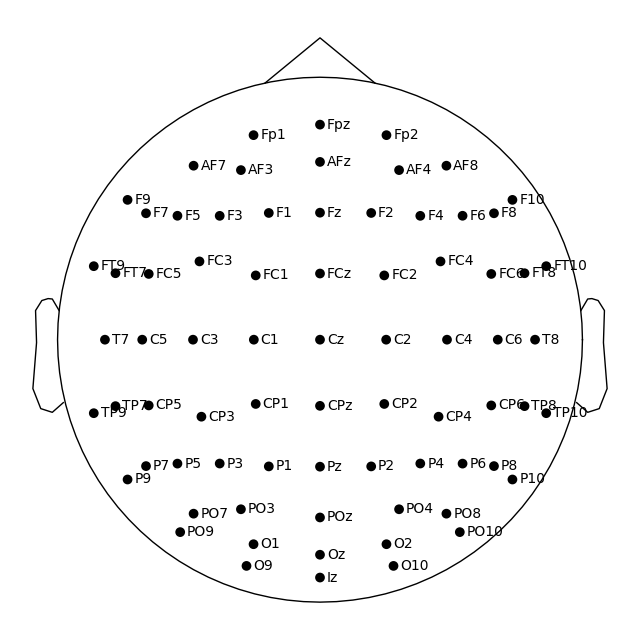•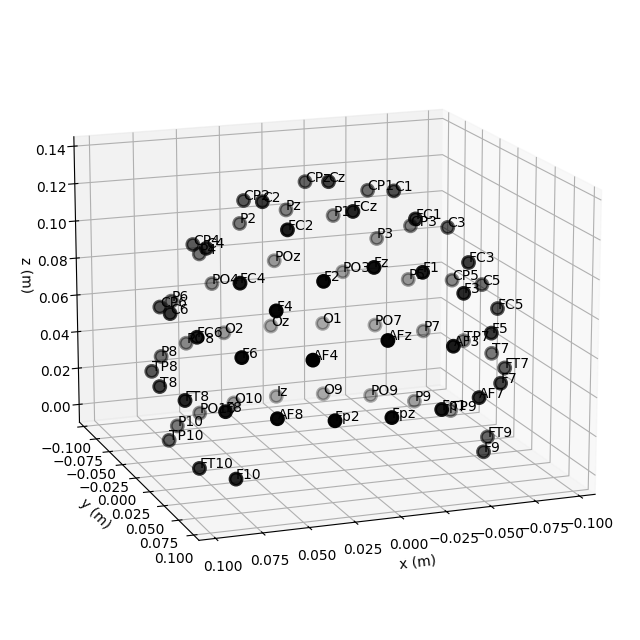```4 duplicate electrode labels found:
T7/T3, T8/T4, P7/T5, P8/T6
Plotting 90 unique labels.
Creating RawArray with float64 data, n_channels=90, n_times=1
Range : 0 ... 0 =      0.000 ...     0.000 secs
4 duplicate electrode labels found:
T7/T3, T8/T4, P7/T5, P8/T6
Plotting 90 unique labels.
Creating RawArray with float64 data, n_channels=90, n_times=1
Range : 0 ... 0 =      0.000 ...     0.000 secs
```

## Controlling channel projection (MNE vs EEGLAB)#

Channel positions in 2D space are obtained by projecting their actual 3D positions onto a sphere, then projecting the sphere onto a plane. Because the `'standard_1020'` montage contains realistic (as opposed to idealized spherical) channel positions, we will use a different montage to demonstrate how channels are projected to 2D:

```biosemi_montage = mne.channels.make_standard_montage('biosemi64')
biosemi_montage.plot(show_names=False)
```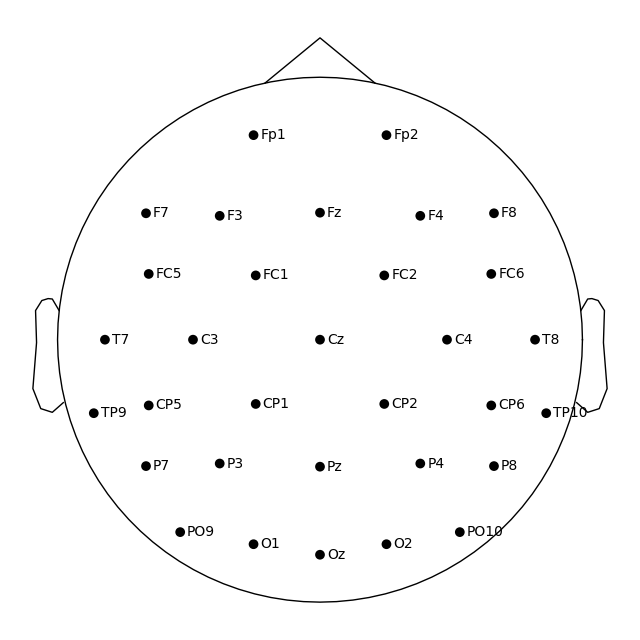```Creating RawArray with float64 data, n_channels=64, n_times=1
Range : 0 ... 0 =      0.000 ...     0.000 secs
```

By default, a sphere with origin at `(0, 0, 0)` (x, y, z coordinates) and radius of `0.095` meters (9.5 cm) is used. You can use a different sphere radius by passing a single value as the `sphere` argument in any function that plots channels in 2D (like `plot` that we use here, but also for example `mne.viz.plot_topomap`):

```biosemi_montage.plot(show_names=False, sphere=0.07)
``````Creating RawArray with float64 data, n_channels=64, n_times=1
Range : 0 ... 0 =      0.000 ...     0.000 secs
```

To change not only the radius, but also the sphere origin, pass a `(x, y, z, radius)` tuple as the `sphere` argument:

```biosemi_montage.plot(show_names=False, sphere=(0.03, 0.02, 0.01, 0.075))
```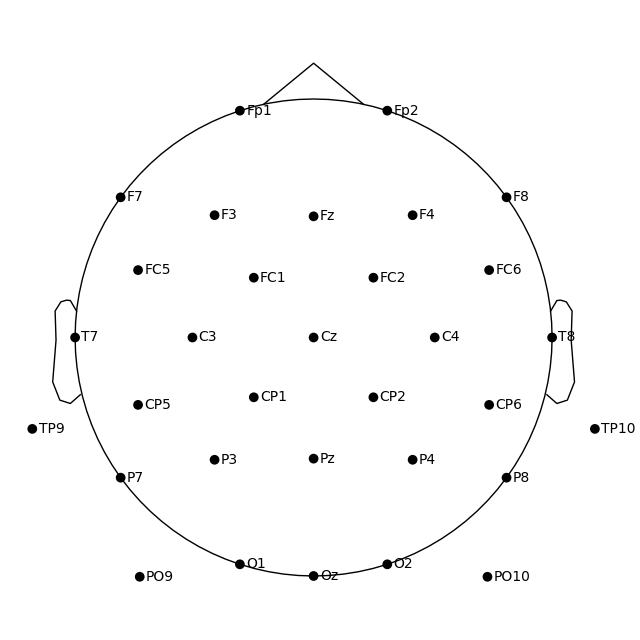```Creating RawArray with float64 data, n_channels=64, n_times=1
Range : 0 ... 0 =      0.000 ...     0.000 secs
```

In MNE-Python, the head center and therefore the sphere center are calculated using fiducial points. This means that the head circle represents the head circumference at the nasion and ear level, and not where it is commonly measured in the 10–20 EEG system (above the nasion at T4/T8, T3/T7, Oz, and Fz). Notice below that by default T7 and Oz are placed within the head circle:

```biosemi_montage.plot()
``````Creating RawArray with float64 data, n_channels=64, n_times=1
Range : 0 ... 0 =      0.000 ...     0.000 secs
```

If you prefer to draw the head circle using 10–20 conventions (which are also used by EEGLAB), you can move the sphere origin a few centimeters up along the z dimension:

```biosemi_montage.plot(sphere=(0, 0, 0.035, 0.094))
```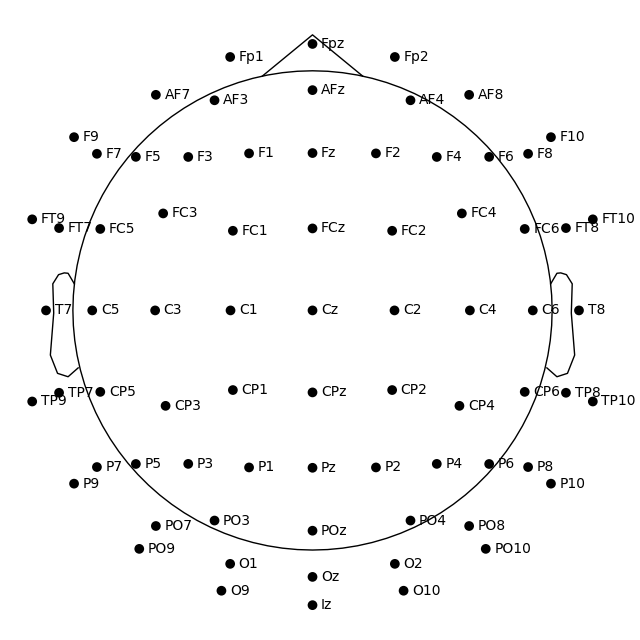```Creating RawArray with float64 data, n_channels=64, n_times=1
Range : 0 ... 0 =      0.000 ...     0.000 secs
```

Alternatively, you can calculate the sphere origin from Oz, Fpz, T3/T7 or T4/T8 channels. This is easier once the montage has been applied to the data and channel positions are in the head space (see this example).

In the sample data, the sensor positions are already available in the `info` attribute of the `Raw` object (see the documentation of the reading functions and `set_montage` for details on how that works). Therefore, we can plot sensor locations directly from the `Raw` object using `plot_sensors`, which provides similar functionality to `montage.plot()`. In addition, `plot_sensors` supports channel selection by type, color-coding channels in various ways (by default, channels listed in `raw.info['bads']` will be plotted in red), and drawing in an existing Matplotlib `Axes` object (so the channel positions can easily be added as a subplot in a multi-panel figure):

```fig = plt.figure()
raw.plot_sensors(ch_type='eeg', axes=ax2d)
raw.plot_sensors(ch_type='eeg', axes=ax3d, kind='3d')
ax3d.view_init(azim=70, elev=15)
```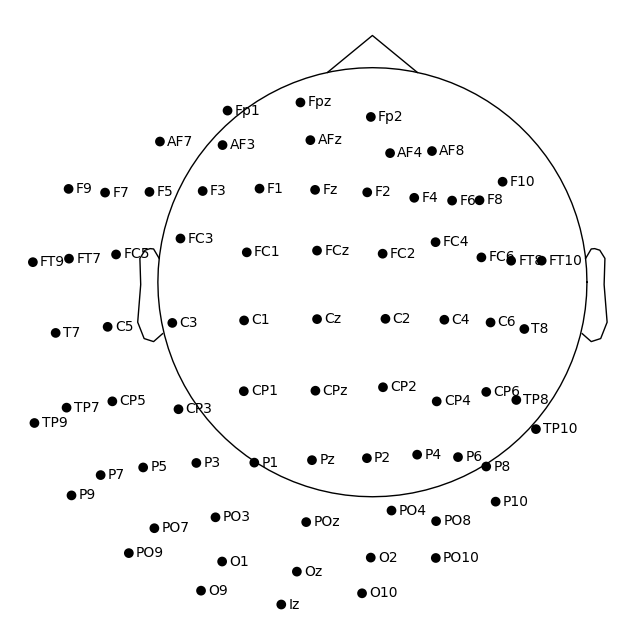The previous 2D topomap reveals irregularities in the EEG sensor positions in the sample dataset — this is because the sensor positions in that dataset are digitizations of actual sensor positions on the head rather than idealized sensor positions based on a spherical head model. Depending on the digitization device (e.g., a Polhemus Fastrak digitizer), you need to use different montage reading functions (see Supported formats for digitized 3D locations). The resulting `montage` can then be added to `Raw` objects by passing it as an argument to the `set_montage` method (just as we did before with the name of the predefined `'standard_1020'` montage). Once loaded, locations can be plotted with the `plot` and saved with the `save` methods of the `montage` object.

Note

When setting a montage with `set_montage`, the measurement info is updated in two places (both `chs` and `dig` entries are updated) – see The Info data structure for more details. Note that `dig` may contain HPI, fiducial, or head shape points in addition to electrode locations.

## Visualizing sensors in 3D surface renderings#

It is also possible to render an image of an MEG sensor helmet using 3D surface rendering instead of matplotlib. This works by calling `mne.viz.plot_alignment`:

```fig = mne.viz.plot_alignment(raw.info, dig=False, eeg=False,
surfaces=[], meg=['helmet', 'sensors'],
coord_frame='meg')
mne.viz.set_3d_view(fig, azimuth=50, elevation=90, distance=0.5)
```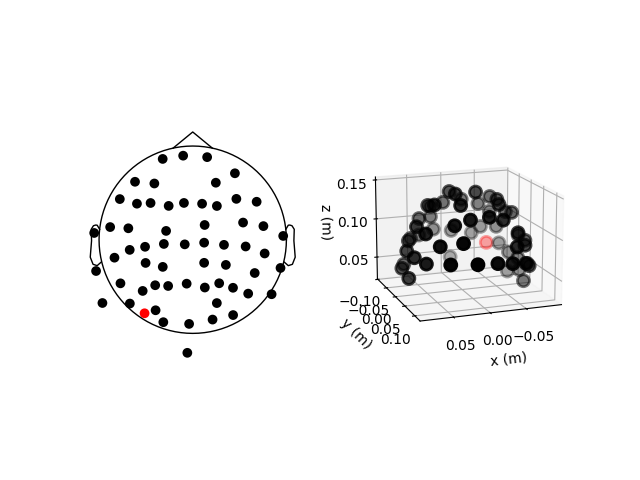```Getting helmet for system 306m
Channel types:: grad: 203, mag: 102
```

Note that `plot_alignment` requires an `Info` object, and can also render MRI surfaces of the scalp, skull, and brain (by passing a dict with keys like `'head'`, `'outer_skull'` or `'brain'` to the `surfaces` parameter). This makes the function useful for assessing coordinate frame transformations. For examples of various uses of `plot_alignment`, see Plotting sensor layouts of EEG systems, Plotting EEG sensors on the scalp, and Plotting sensor layouts of MEG systems.

## Working with layout files#

Similar to montages, many layout files are included with MNE-Python. They are stored in the `mne/channels/data/layouts` folder:

```layout_dir = os.path.join(os.path.dirname(mne.__file__),
'channels', 'data', 'layouts')
print('\nBUILT-IN LAYOUT FILES')
print('=====================')
print(sorted(os.listdir(layout_dir)))
```
```BUILT-IN LAYOUT FILES
=====================
['CTF-275.lout', 'CTF151.lay', 'CTF275.lay', 'EEG1005.lay', 'EGI256.lout', 'KIT-125.lout', 'KIT-157.lout', 'KIT-160.lay', 'KIT-AD.lout', 'KIT-AS-2008.lout', 'KIT-UMD-3.lout', 'Neuromag_122.lout', 'Vectorview-all.lout', 'Vectorview-grad.lout', 'Vectorview-grad_norm.lout', 'Vectorview-mag.lout', 'biosemi.lay', 'magnesWH3600.lout']
```

The file formats (and therefore file extensions) of the built-in layout and montage files vary considerably (because manufacturers like to use different conventions). However, the montage and layout loading functions in MNE-Python take the filename without its extension so you do not have to keep track of which file format is used by which manufacturer.

To load a layout file, use the `mne.channels.read_layout` function and provide the filename without its file extension. You can then visualize the layout using its `plot` method or equivalently passing the layout to `mne.viz.plot_layout`:

```biosemi_layout = mne.channels.read_layout('biosemi')
biosemi_layout.plot()  # same result as mne.viz.plot_layout(biosemi_layout)
```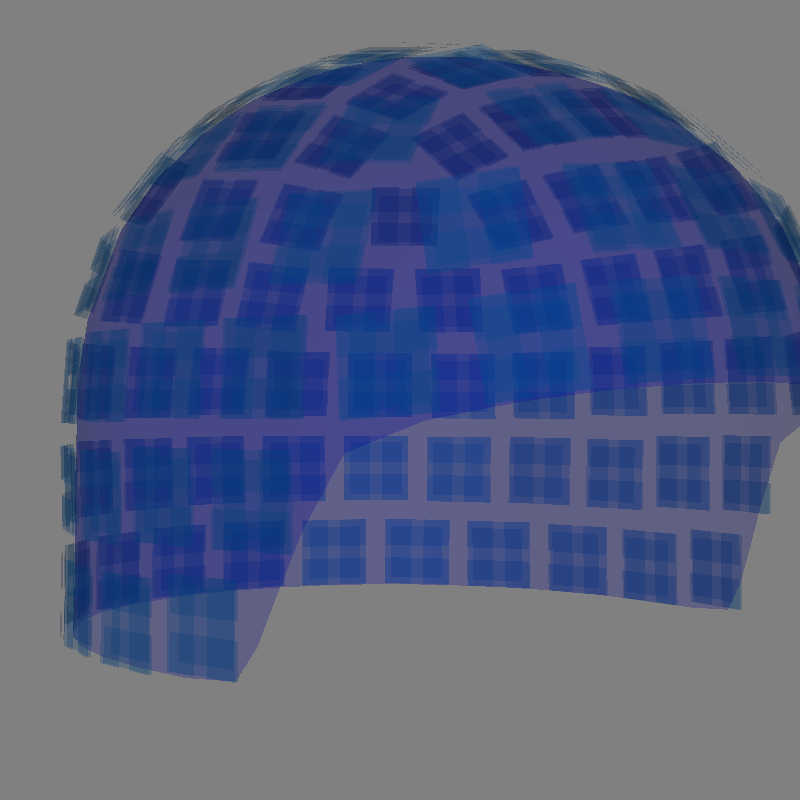Similar to the `picks` argument for selecting channels from `Raw` objects, the `plot` method of `Layout` objects also has a `picks` argument. However, because layouts only contain information about sensor name and location (not sensor type), the `plot` method only supports picking channels by index (not by name or by type). In the following example, we find the desired indices using `numpy.where`; selection by name or type is possible with `mne.pick_channels` or `mne.pick_types`.

```midline = np.where([name.endswith('z') for name in biosemi_layout.names])
biosemi_layout.plot(picks=midline)
```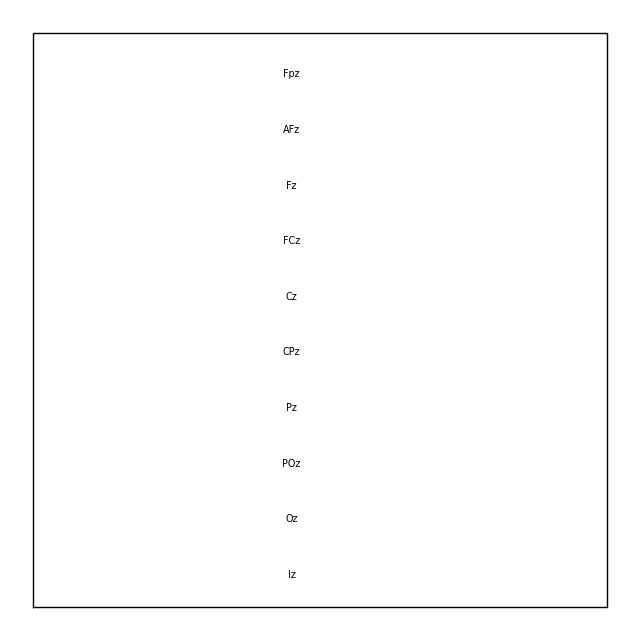If you have a `Raw` object that contains sensor positions, you can create a `Layout` object with either `mne.channels.make_eeg_layout` or `mne.channels.find_layout`.

```layout_from_raw = mne.channels.make_eeg_layout(raw.info)
# same result as mne.channels.find_layout(raw.info, ch_type='eeg')
layout_from_raw.plot()
```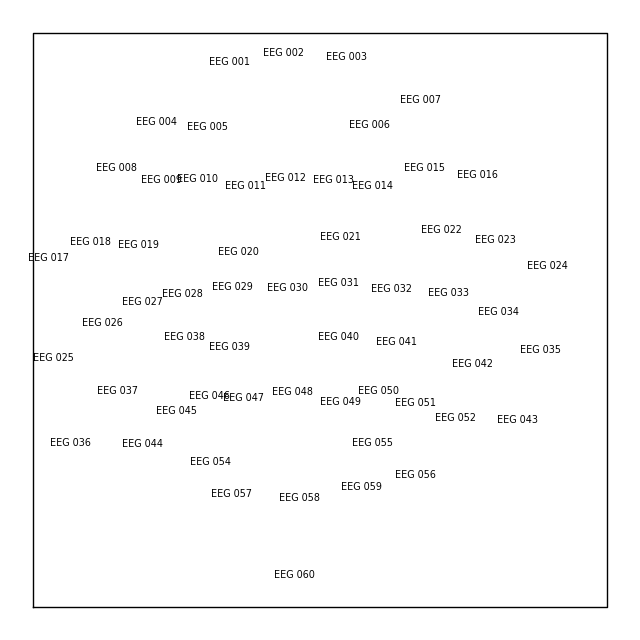Note

There is no corresponding `make_meg_layout()` function because sensor locations are fixed in an MEG system (unlike in EEG, where sensor caps deform to fit snugly on a specific head). Therefore, MEG layouts are consistent (constant) for a given system and you can simply load them with `mne.channels.read_layout` or use `mne.channels.find_layout` with the `ch_type` parameter (as previously demonstrated for EEG).

All `Layout` objects have a `save` method that writes layouts to disk as either `.lout` or `.lay` formats (inferred from the file extension contained in the `fname` argument). The choice between `.lout` and `.lay` format only matters if you need to load the layout file in some other application (MNE-Python can read both formats).

Total running time of the script: ( 0 minutes 18.012 seconds)

Estimated memory usage: 494 MB

Gallery generated by Sphinx-Gallery